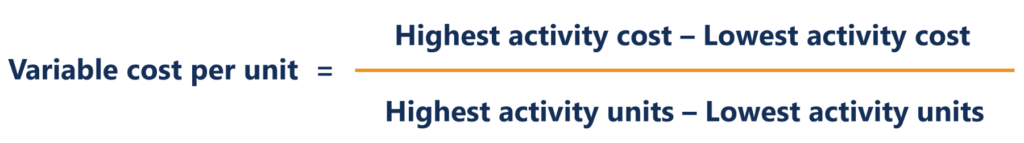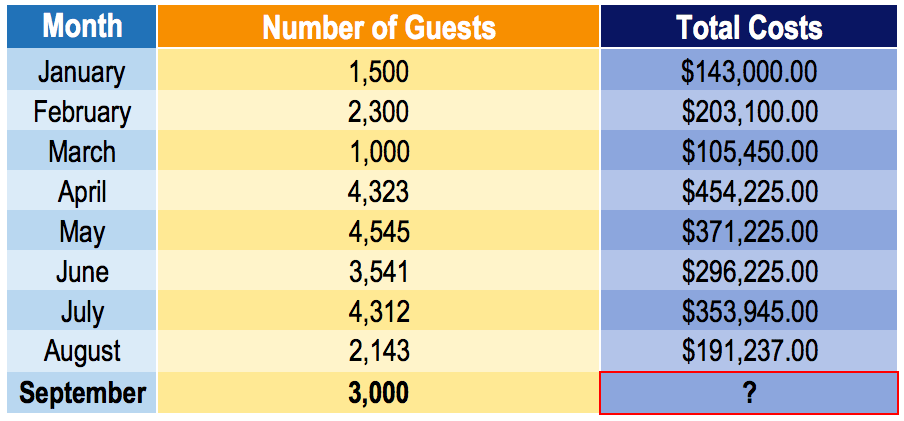# High-Low Method

A technique used in cost accounting to split mixed costs into fixed and variable costs

## What is High-Low Method?

In cost accounting, the high-low method is a technique used to split mixed costs into fixed and variable costs. Although the high-low method is easy to apply, it is seldom used, because it can distort costs, due to its reliance on two extreme values from a given data set.

### High-Low Method Formula

The formula for developing a cost model using the high-low method is as follows:Once the variable cost per unit is determined:

Fixed cost = Highest activity cost – (Variable cost per unit x Highest activity units)

or

Fixed cost = Lowest activity cost – (Variable cost per unit x Lowest activity units)

The resulting cost model after using the high-low method would be as follows:

Cost model = Fixed cost + Variable cost x Unit activity

### Example of the High-Low Method

The manager of a hotel would like to develop a cost model to predict the future costs of running the hotel. Unfortunately, the only data that is available to him is the level of activity (number of guests) in a given month and the total costs incurred in each month. Being a new hire at the company, the manager assigns you the task of anticipating the costs that would be incurred in the following month (September).

He anticipates that the number of guests in September will be 3,000. Given the dataset below, develop a cost model and predict the costs that will be incurred in September.Using the high-low method:Note: It is important to choose the high-low values from the units (i.e., number of guests) and not the total costs. The number of units drives the total costs. For example, notice that the highest total cost is \$454,255, which corresponds to an activity level of 4,323. However, the correct high-low values are from the independent variable (the variable that predicts the costs). In such a case, the high and low will be 4,545 number of guests in May (total costs: \$371,225) and 1,500 number of guests in January (total costs: \$143,000).

Given the variable cost per number of guests, we can now determine our fixed costs.

Using the high activity cost:

Fixed cost = \$371,225 – (\$74.97 x 4,545) = \$30,486.35

Using the low activity cost:

Fixed cost = \$105,450 – (\$74.97 x 1,000) = \$30,480

Using either the high or low activity cost should yield approximately the same fixed cost value. Note that our fixed cost differs by \$6.35 depending on whether we use the high or low activity cost. It is a nominal difference and choosing either fixed cost for our cost model will suffice.

Cost model for hotel = \$30,480 + \$74.97 x No. of guests

Therefore, the anticipated costs for September would be:

September costs = \$30,480 + \$74.97 x 3,000 = \$255,390

### Advantages of the High-Low Method

The high-low method is an easy way to segregate fixed and variable costs. By only requiring two data values and some algebra, cost accountants can quickly and easily determine information about cost behavior. Also, the high-low method does not use or require any complex tools or programs.

Due to the simplicity of using the high-low method to gain insight into the cost-activity relationship, it does not take into consideration small details such as variation in costs. The high-low method assumes that fixed and unit variable costs are constant, which is not the case in real life. Because it uses only two data values in its calculation, variations in costs are not captured in the estimate.

Another drawback of the high-low method is the ready availability of better cost estimation tools. For example, the least-squares regression is a method that takes into consideration all data points and creates an optimized cost estimate. It can be easily and quickly used to yield significantly better estimates than the high-low method.

CFI is the official provider of the global Financial Modeling & Valuation Analyst (FMVA)™ certification program for anyone looking to become a world-class financial analyst. To keep advancing your career, the additional CFI resources below will be useful:

• Cost Behavior Analysis
• Cost Method
• Marginal Cost Formula
• Financial Modeling Templates